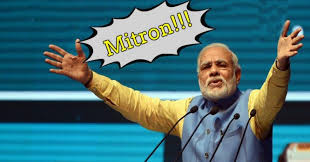# Achhe Din

Problem:-Modiji does not like duplicates in his life. However, he has found a piece of paper where multiple integers are present. He observed and found out that all elements occurred thrice there except one element, which occurs just once.

He wishes to find that rogue integer. Help him find the element that occurs once.

Input Format

The first line contains a single integer, containing the number of testcases, T. Each test case contains 2 lines. The first line contains 1 integer, N. The next line contains N space separated integers.

Output Format

The output consists of just T lines. Each line consists of 1 integer, that is, the element that occurs once.

Constraints

1<=T<=5

1<=N<=10^5

1<=arr[i]<=10^6

Sample Input
`171 2 2 1 2 1 4`
Sample Output
`4`
Time Limit: 1
Memory Limit: 256
Source Limit:

Code:-

#include<stdio.h>
int main()
{
int t;
scanf(“%d”,&t);
while(t–)
{
long int n,i;
scanf(“%ld”,&n);
long long int a={0},element;
for(i=0;i<n;i++)
{
scanf(“%lld”,&element);
a[element]++;
}
for(i=1;i<=1000000;i++)
{
if(a[i]==1)
{
printf(“%lldn”,i);
}
}
}
return 0;
}